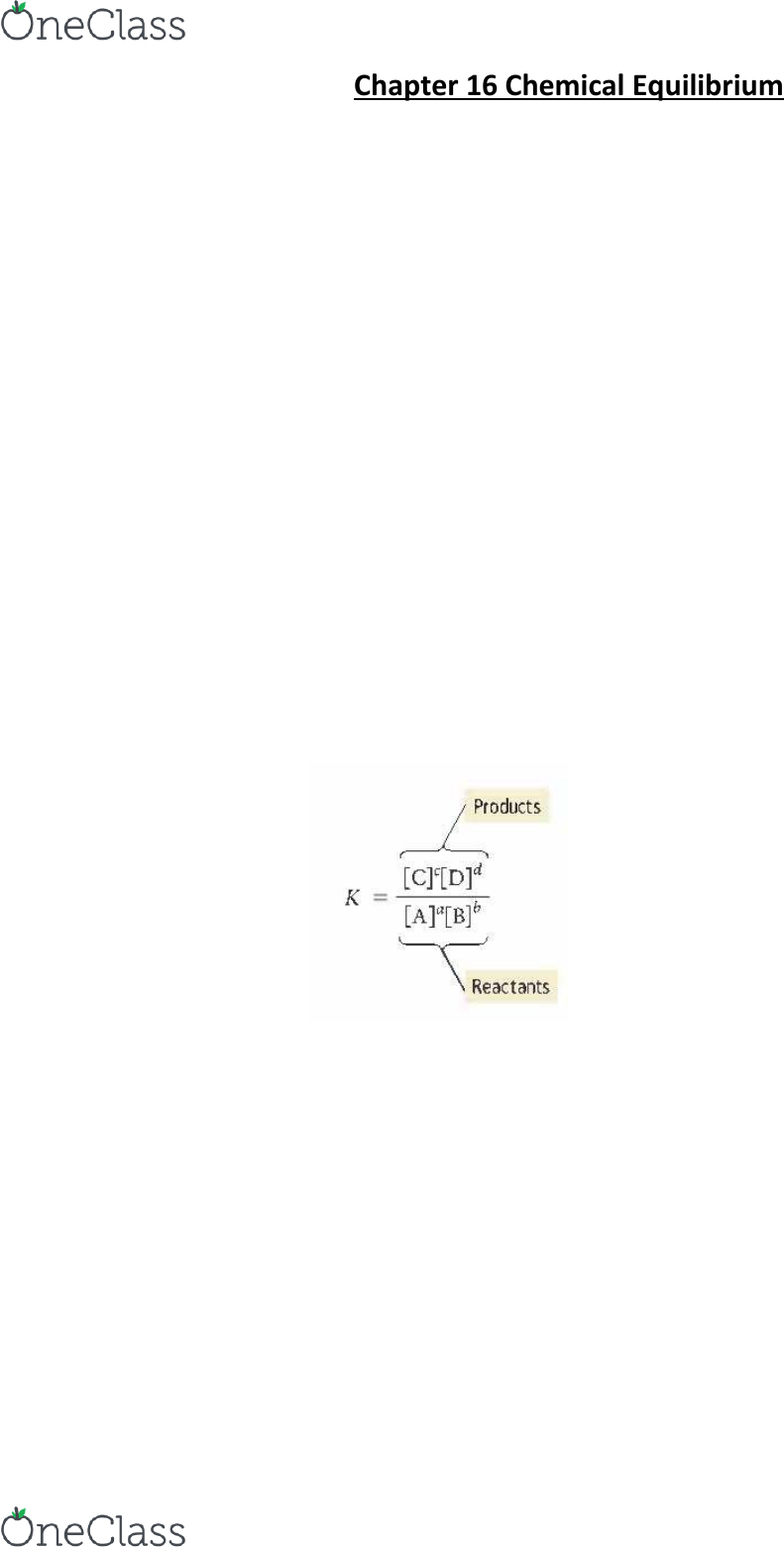Textbook Notes (290,000)
US (110,000)
UC-Irvine (3,000)
CHEM (200)
CHEM 1C (20)
Chapter 16

# CHEM 1C Chapter Notes - Chapter 16: Inert Gas, Endothermic Process, Reaction Quotient

Department
Chemistry
Course Code
CHEM 1C
Professor
Eric Potma
Chapter
16

Page:
of 3Chapter 16 Chemical Equilibrium
16.1 Fetal Hemoglobin and Equilibrium
- double arrow indicates reaction can occur in both directions and therefore reach equilibrium
- Large K means high concentration of products and low reactants.
- Small K means low concentration products and high concentration reactants.
- Equilibrium Constant K is a measure of how far a reaction proceeds
- Hemoglobin binds oxygen when the surrounding oxygen concentration is high, but it releases oxygen when
the surrounding oxygen concentration is low
16.2 The Concept of Dynamic Equilibrium
- Reversible = a reaction that can proceed in both the forward and the reverse direction
-Dynamic Equilibrium = is the condition in which the rate of the forward reaction equals the rate of the
reverse reaction
- concentration of products and reactants remain constant but they do not equal each other
16.3 The Equilibrium Constant (K)
- quantify relative concentrations with quantity called Equilibrium Constant (k)
- Equilibrium constant (K) = = law of mass action
Expressing Equilibrium Constant for Chemical Reactions
oExamine balanced chemical equation and apply law of mass action
The Significance of the Equilibrium Constant
oWhen equilibrium constant is large, forward reaction is favored
oWhen equilibrium constant is small, the reverse reaction is favored
Relationship between the Equilibrium Constant and the Chemical Equation
1. If we reverse the equation, we invert the equilibrium constant
2. If we multiply the coefficients in the equation by a factor, we raise the equilibrium constant to
the same factor
find more resources at oneclass.com
find more resources at oneclass.com
3. If we add two or more individual chemical equations to obtain an overall reaction, we multiply
the corresponding equilibrium constants by each other to obtain the overall equilibrium
constant
16.4 Expressing the Equilibrium Constant in Terms of Pressure
- for gaseous reactions, the partial pressure of a particular gas is proportional to its concentration
- can express equilibrium constant in terms of the partial pressures of the reactants and products
- The expression for Kp takes the form of the expression for Kc, except that we use the partial pressure of each
gas in place of its concentration
Units of K
oNo units because they are just ratios so the units cancel out
16.5 Heterogeneous Equilibria: Reactions Involving Solids and Liquids
- Concentration of a solid is constant, because it does not expand to fill its container.
- Concentration of a solid depends only on its density.
- Pure solids and liquids are not included in equilibrium expression
16.6 Calculating the Equilibrium Constant from Measured Equilibrium Concentrations
- most direct way to obtain equilibrium constant of reaction is to measure concentrations at equilibrium
- most cases need only know the initial of reactant(s) and equilibrium of any one reactant or product
- other equilibrium concentrations can be determined from stoichiometry of the reaction
- Initial Change Equilibrium = ICE Table. Last row is sum of the two rows above it
16.7 The Reaction Quotient: Predicating the Direction of Change
-A reaction mixture at equilibrium contains both reactants and products, how to predict direction
- reaction quotient (Qc) = the ratio – at any point in the reaction – of the concentrations of the products raised
to their stoichiometric coefficients divided by the concentrations of the reactants raised to their stoichiometric
coefficients.
- gases use partial pressures instead of concentrations.
- at a given temperature, equilibrium constant has only one value. Reaction quotient depends on many values
- mixture: only reactants Qc = 0, mixture: only products Qc = ∞, both reactants and products Qc = 1
find more resources at oneclass.com
find more resources at oneclass.com
- The value of Q relative to K is a measure of the progress of the reaction toward equilibrium.
- At equilibrium the reaction quotient is equal to the equilibrium constant
- Q < K Reaction goes to the right (toward products)
- Q > K Reaction goes to the left (toward reactants)
- Q = K Reaction is at equilibrium
16.8 Finding Equilibrium Concentrations
- finding equilibrium concentrations from equilibrium constant & (1) all but one concentration, (2) only initial
Finding Equilibrium Concentrations from the Equilibrium Constant and All but One of the Equilibrium
Concentrations of the Reactants and Products
Finding Equilibrium Concentrations from the Equilibrium Constant and Initial Concentrations or
Pressures
oSetup ICE table with given initial concentrations and represent unknown change in [A] with x
oSetup equilibrium constant expression as an expression and solve for x
oSame method for partial pressures as opposed to concentrations
Simplifying Approximations in Working Equilibrium Problems
oIf it proceeds to the right, initial concentration is very large and x will be small relative to initial
oMethod of successive approximation = solve for x as if it were small then substitute back in
oX is a small approximation so that it makes very little change to the equation
16.9 Le Châtelier’s Principle: How a System at Equilibrium Responds to Disturbances
- Le Châtelier’s principle = When a chemical system at equilibrium is disturbed, the system shifts in a direction
that minimizes the disturbance.
- reaction shifts in a direction that minimizes the disturbance
The Effect of a Concentration Change on Equilibrium
oIncreasing concentration of reactants/ decreasing concentration of products, reaction shifts to
the right
oIncreasing concentration of products decreasing/ concentration of reactants, reaction shifts to
the left
The Effect of a Volume (or Pressure) Change on Equilibrium
oDecreasing volume shifts reaction in direction that has fewer moles of gas particles
oIncreasing volume shifts reaction in direction that has greater moles of gas particles
oAdding inert gas to fixed volume has no effect on equilibrium
find more resources at oneclass.com
find more resources at oneclass.com